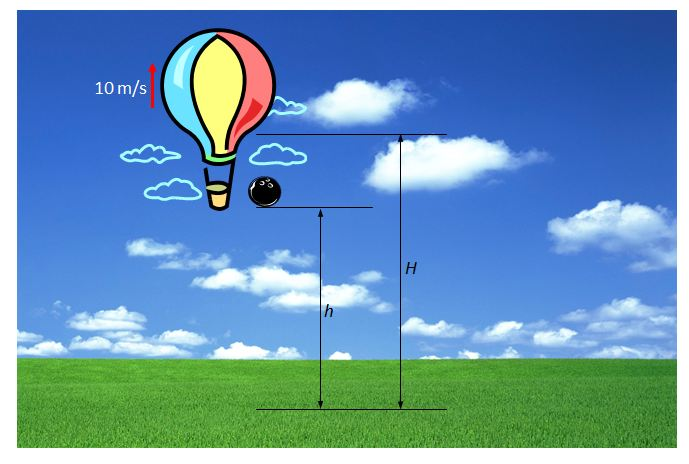# Free FallAs shown above, a black bowling ball is dropped from a hot-air balloon which is ascending straight upwards at a constant speed of $10$ m/s. The height of the bowling ball at the moment of the drop is $h,$ and its highest height before it fell on the ground $3$ seconds after the drop is $H.$ What is the ratio $h:H$?

Gravitational acceleration is $10 \text{ m/s}^2$ and air resistance is negligible.

×